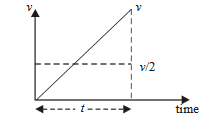#### An athlete in the olympic games covers a distance of 100 m in 10 s. His kinetic energy can be estimated to be in the range Option 1)Option 2)Option 3)Option 4)As we discussed in

Kinetic energy -- whereinkinetic Energy is never negativeInitial velocity = 0

Final velocity vf.a = 2 m/s2

Maximum speed = at = 20 m/s2

Maximum K.E.(Assume m = 50 kg)If it run with constant velocity v = 10 m/s

Then K.E.So it lies between 2500 to 10,000 J.

Correct option is 1

Option 1)This is the correct option.

Option 2)This is an incorrect option.

Option 3)This is an incorrect option.

Option 4)This is an incorrect option.